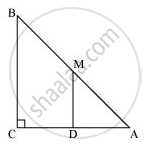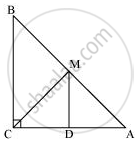# Abc is a Triangle Right Angled at C. a Line Through the Mid-point M of Hypotenuse Ab and Parallel to Bc Intersects Ac at D. Show that - Mathematics

ABC is a triangle right angled at C. A line through the mid-point M of hypotenuse AB and parallel to BC intersects AC at D. Show that

(i) D is the mid-point of AC

(ii) MD ⊥ AC

(iii) CM = MA = 1/2AB

#### Solution(i) In ΔABC,

It is given that M is the mid-point of AB and MD || BC.

Therefore, D is the mid-point of AC. (Converse of mid-point theorem)

(ii) As DM || CB and AC is a transversal line for them, therefore,

∠MDC + ∠DCB = 180º (Co-interior angles)

∠MDC + 90º = 180º

∠MDC = 90º

∴ MD ⊥ AC

(iii) Join MC.In ΔAMD and ΔCMD,

AD = CD (D is the mid-point of side AC)

DM = DM (Common)

∴ ΔAMD ≅ ΔCMD (By SAS congruence rule)

Therefore, AM = CM (By CPCT)

However, AM = 1/2AB (M is the mid-point of AB)

Therefore, it can be said that

CM = AM = 1/2AB

Concept: The Mid-point Theorem
Is there an error in this question or solution?

#### APPEARS IN

NCERT Class 9 Maths